## Wednesday, December 26, 2018

2013
(November)
MATHEMATICS
(Major)
Course: 501
(Logic and Combinatorics, and Analysis – III)
Full Marks: 80
Pass Marks: 32
Time: 3 hours
The figures in the margin indicate full marks for the questions
(A) Logic and Combinatorics
(Marks: 35)

1. (a) Define truth function. 1
(b) Let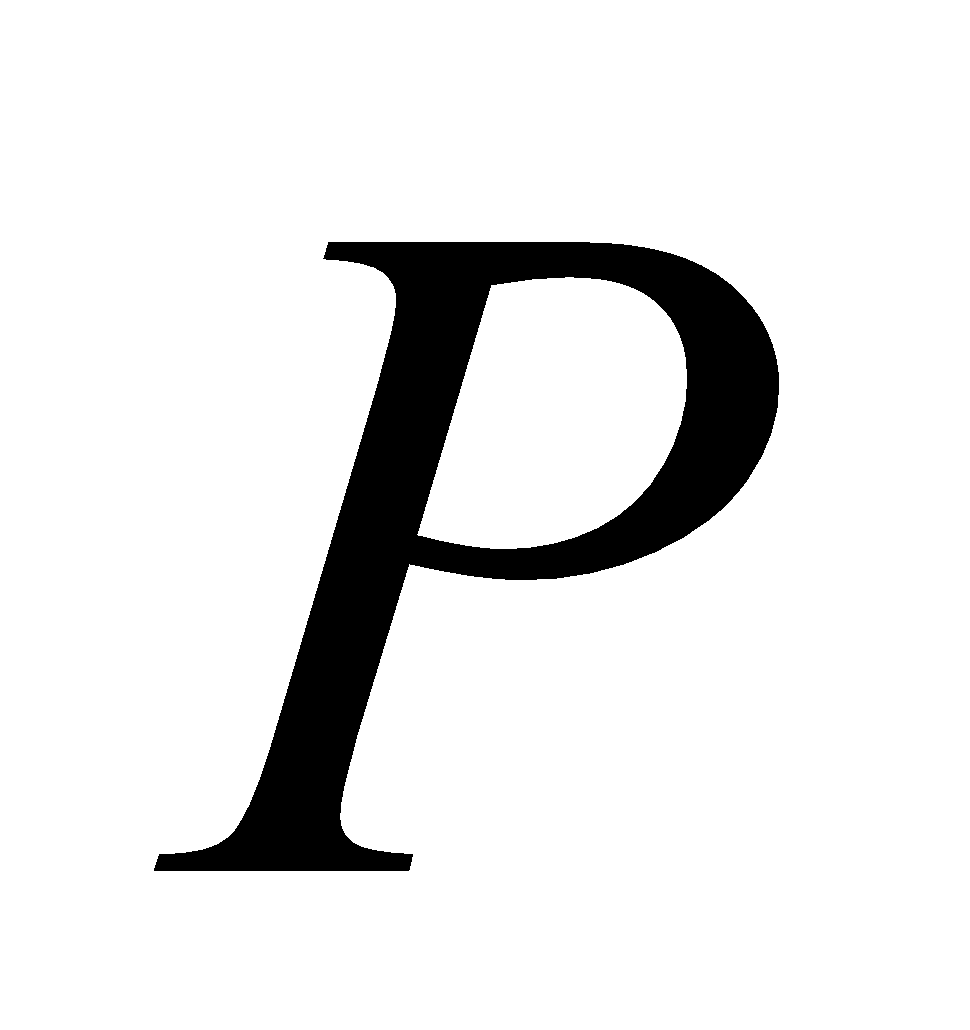be ‘it is cold’ and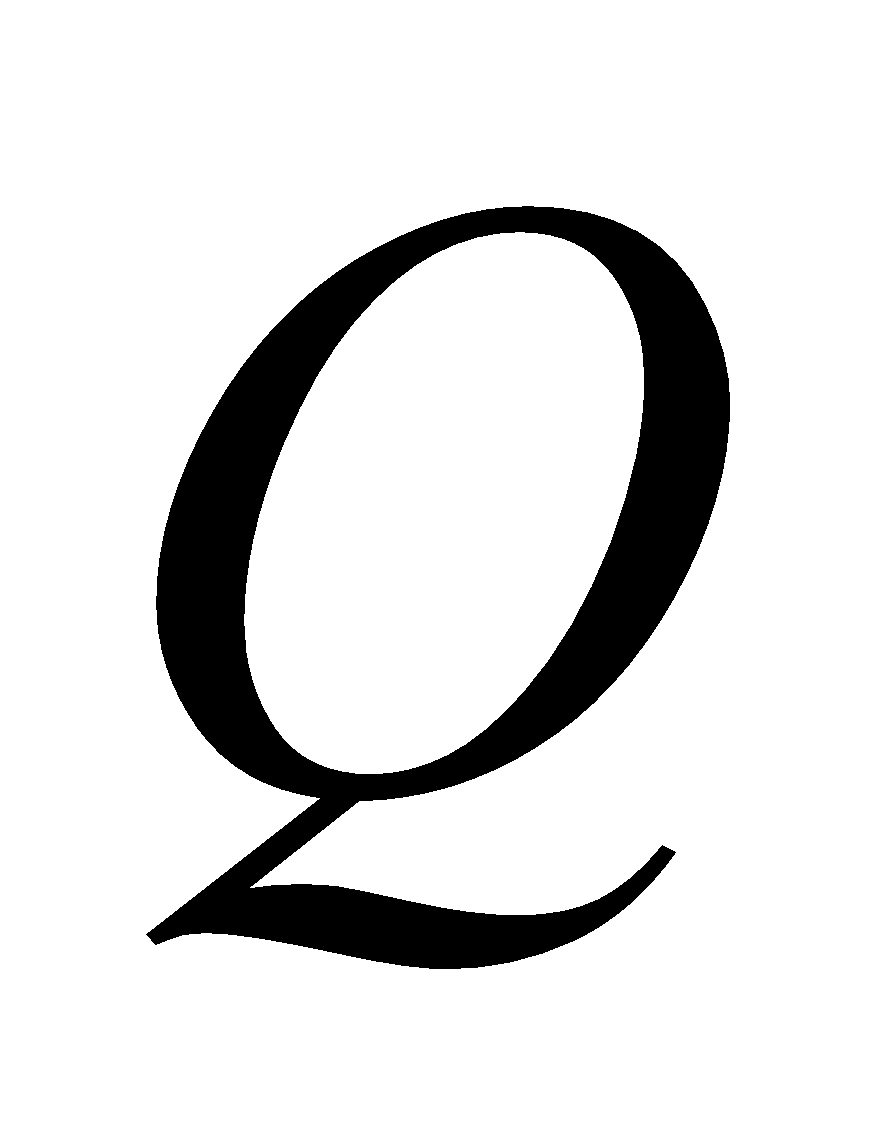be ‘it is raining’. Give verbal sentence which describes each of the following: 2
1.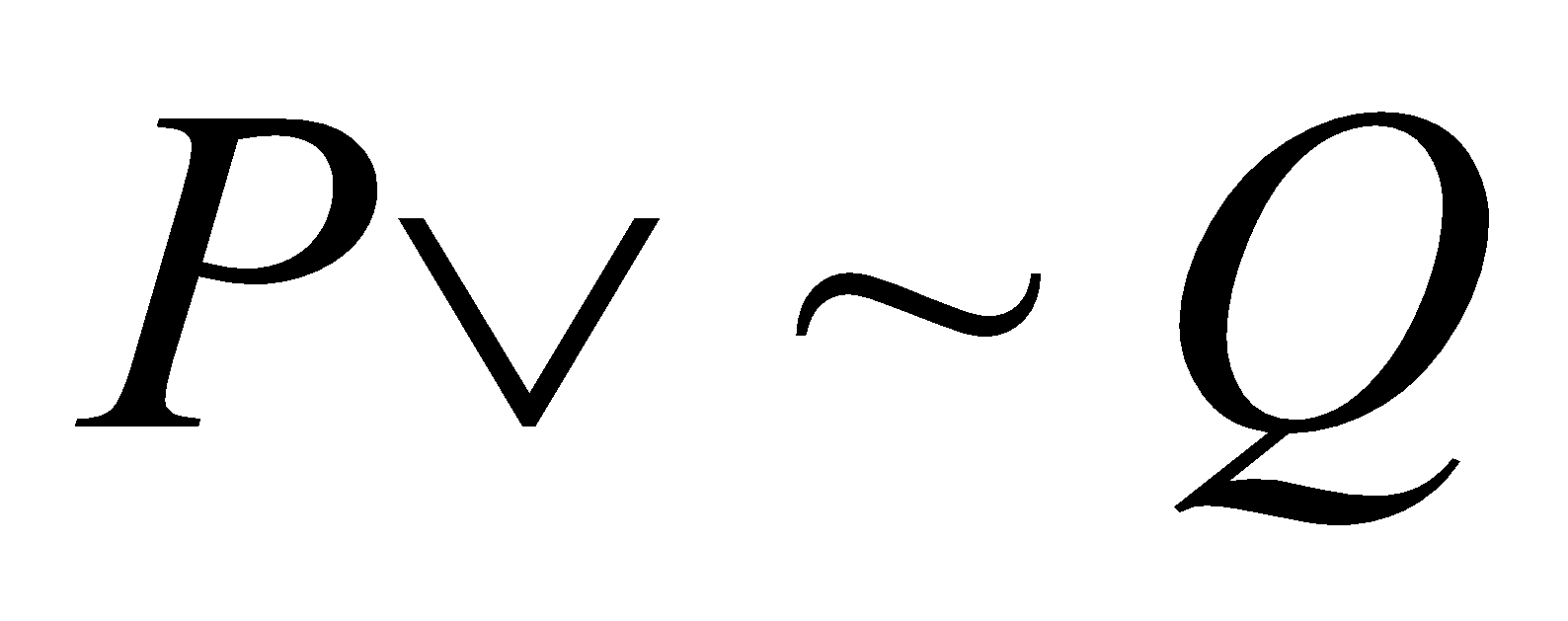2.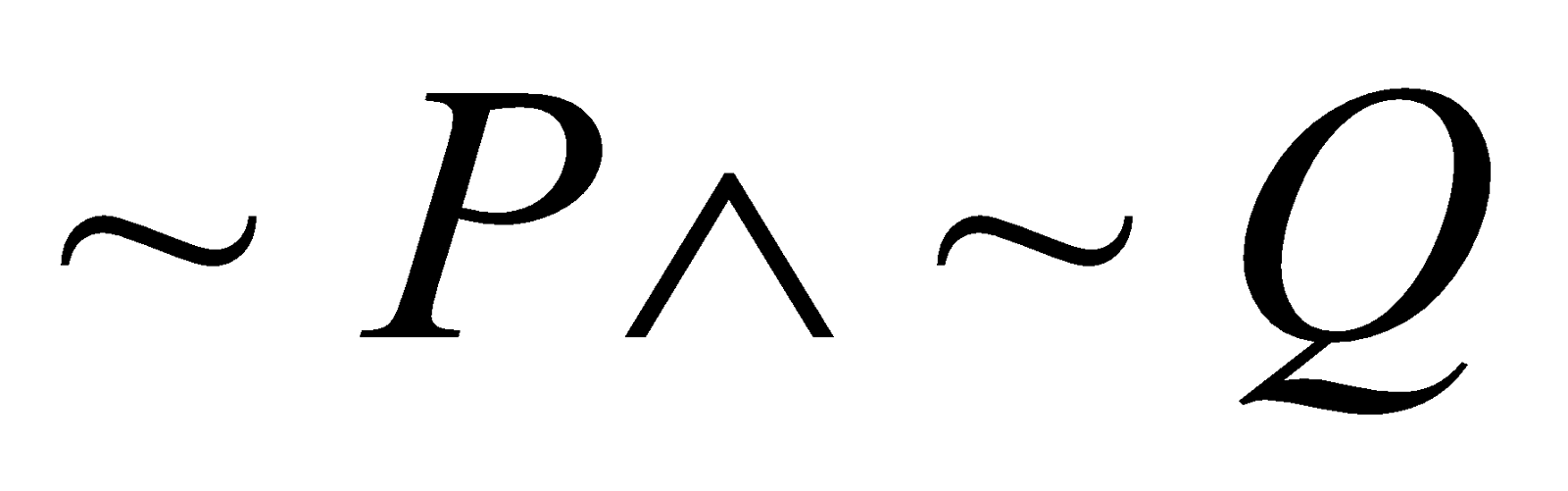(c) Construct the truth table for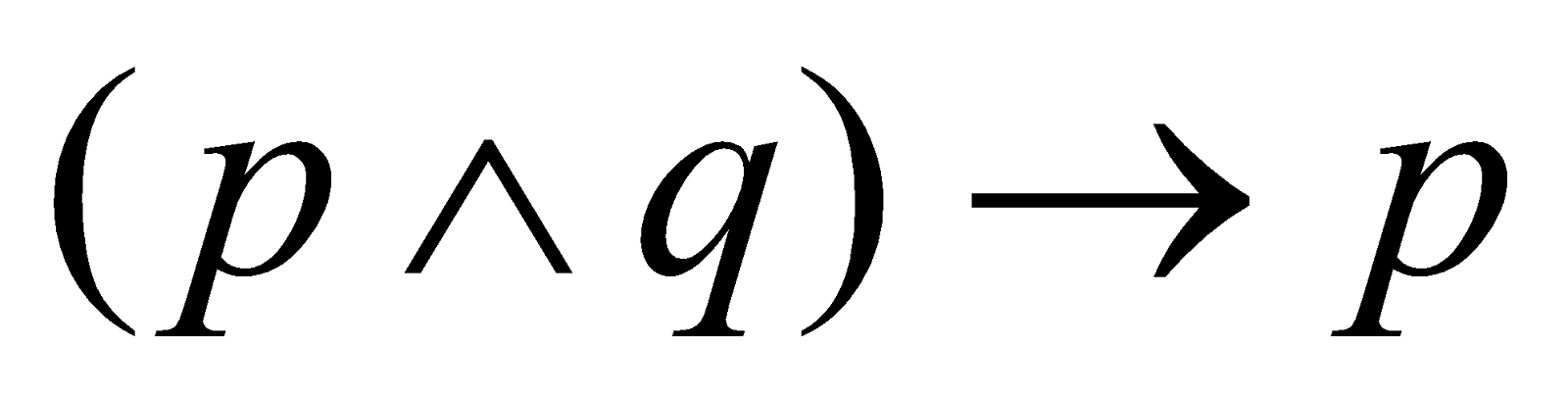. State whether it is a tautology or not. 3
(d) Prove that every truth function can be generated by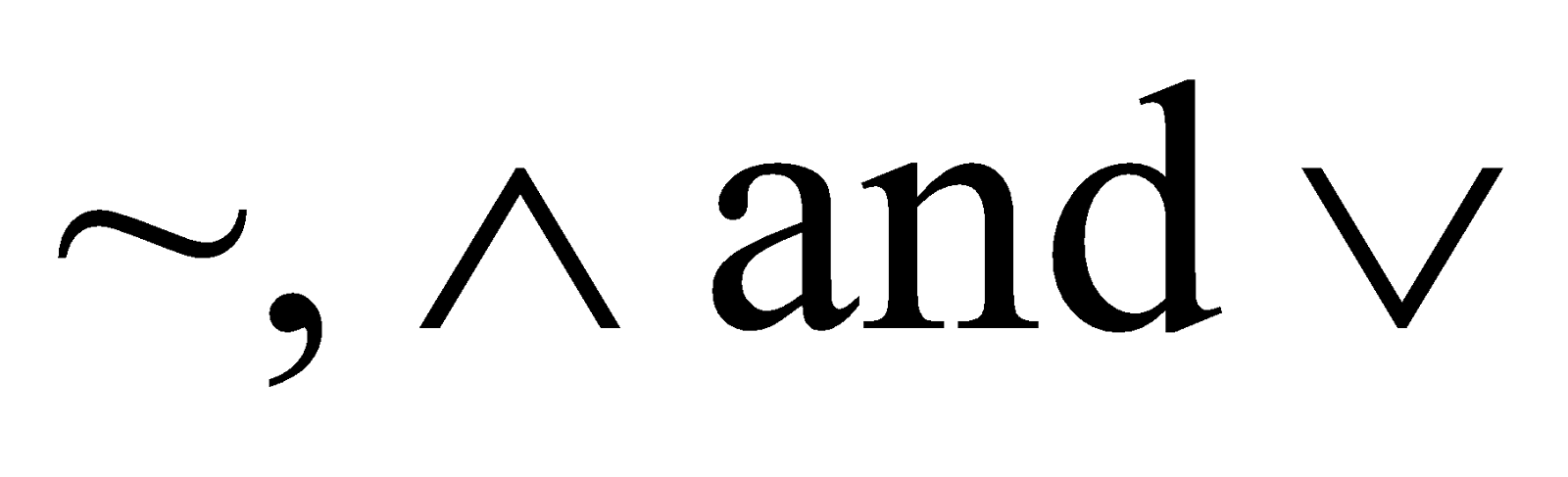. Can you generate a truth function by using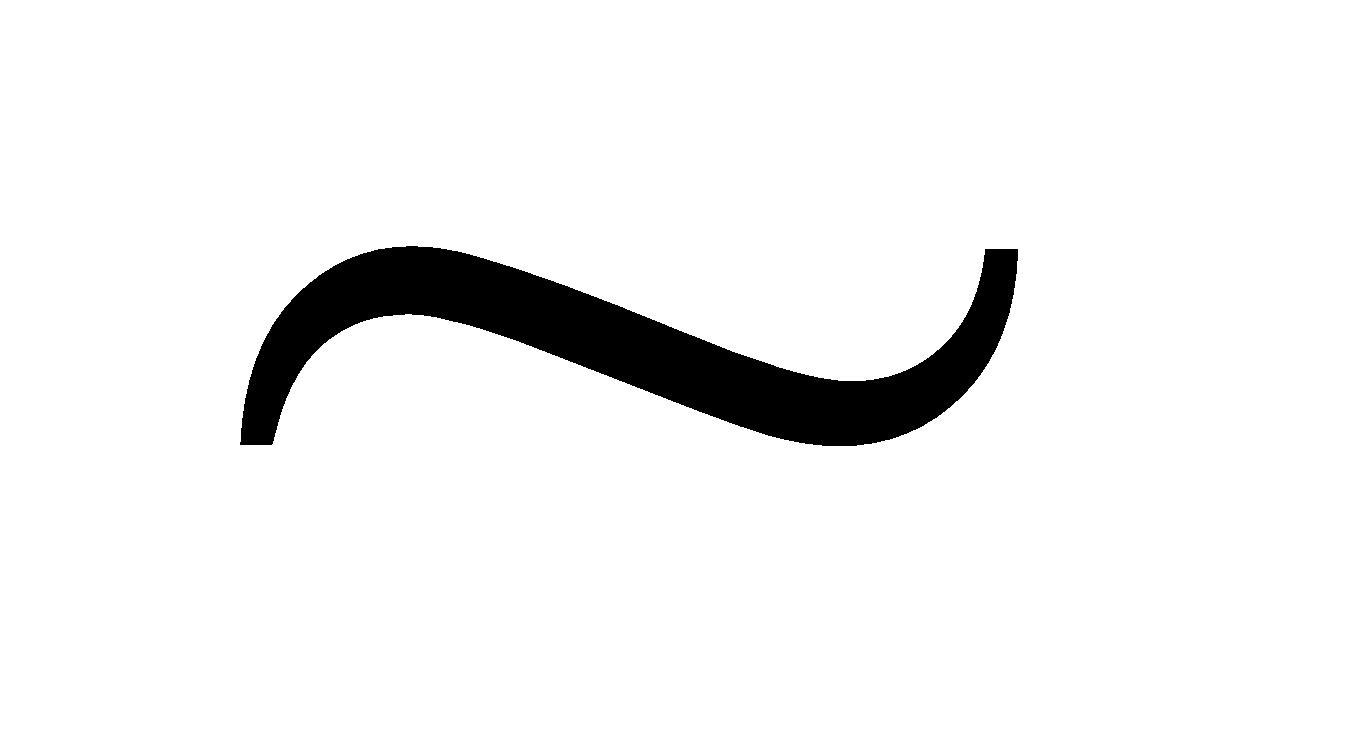and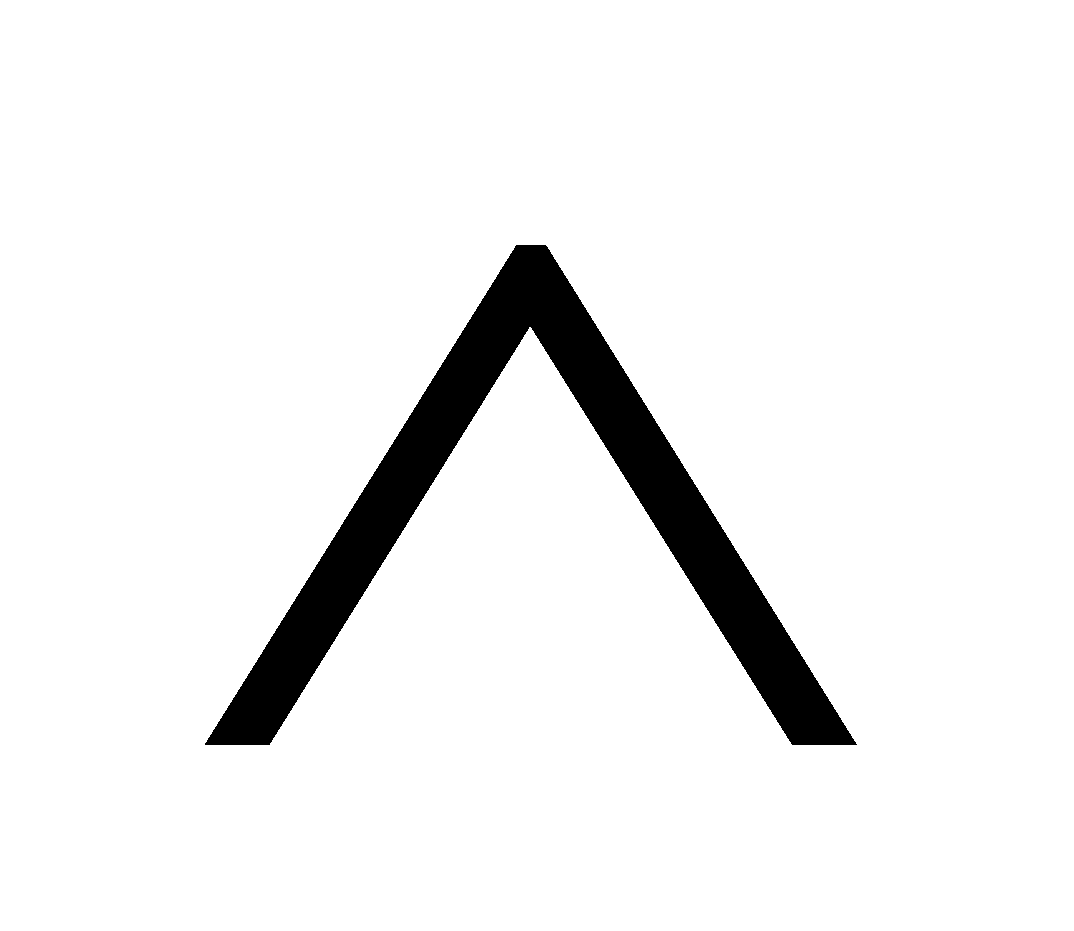only? 4
Or
Give the arithmetic representation of the form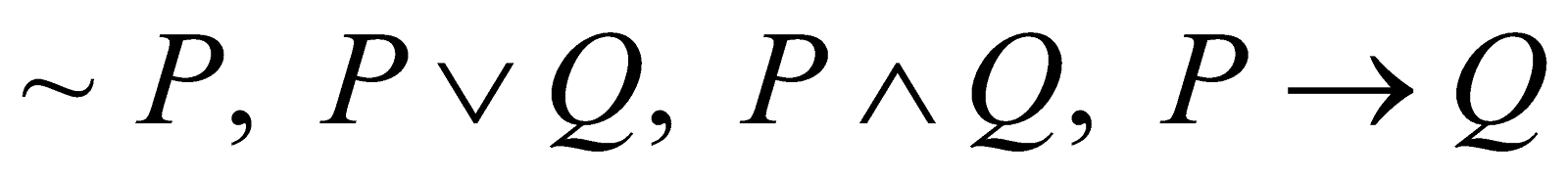. Also show that2. (a) What do you mean by equivalent statements? 1
(b) Write the rule p and rule t. 2
(c) Translate into symbols 3
1. Not all birds can fly.
2. Anyone can do it.
3. Some people are intelligent.
(d) Derive any one of the following: 4
1. Everyone who buys a ticket receives a prize. Therefore, if there is no prize, there nobody buys ticket.
2. All men are mortal. Ram is a man. Hence Ram is mortal.
3. (a) State the Pascal’s identity. 1
(b) Find the coefficient of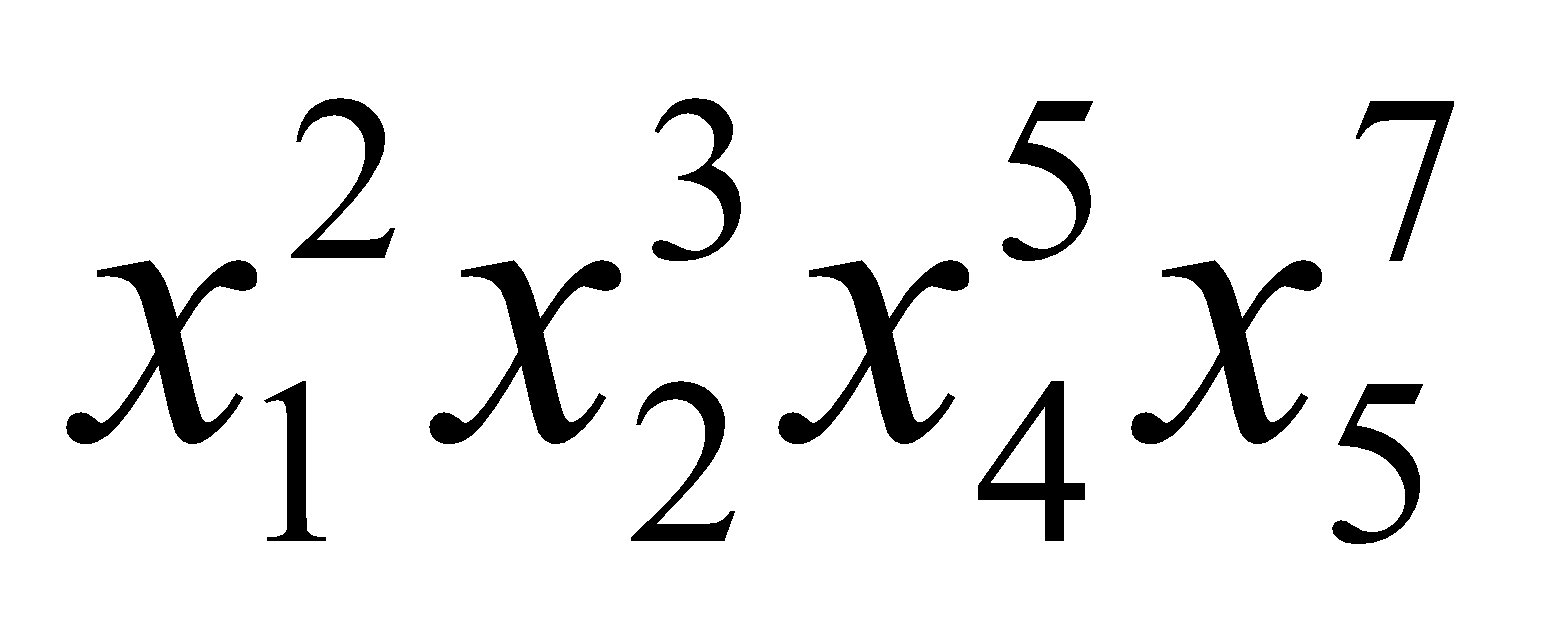in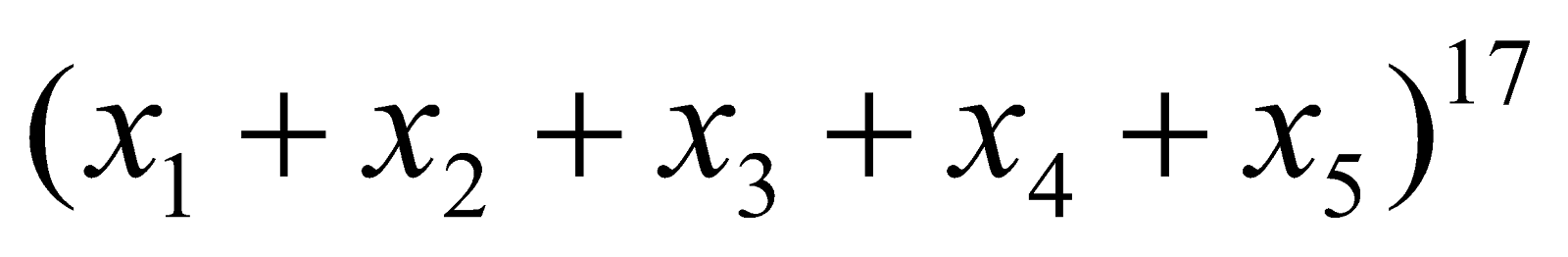. 2
(c) Define Ramsey number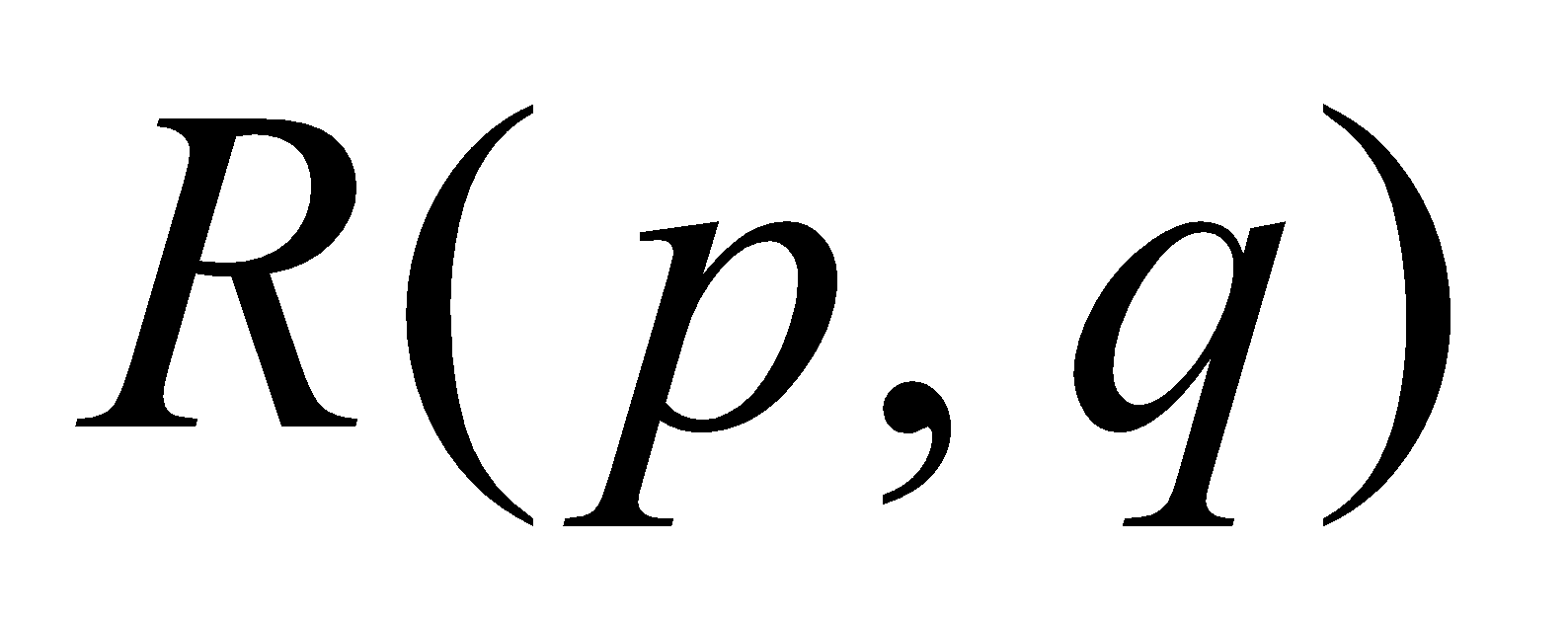. Prove that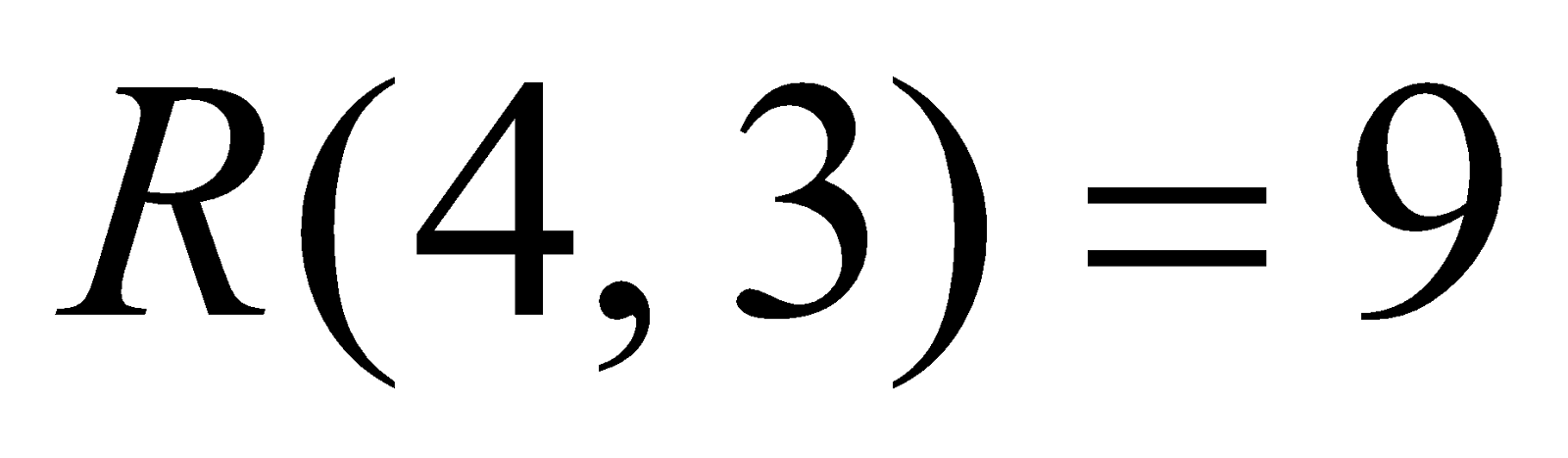. 4
Or
Define Catalan numbers. Prove that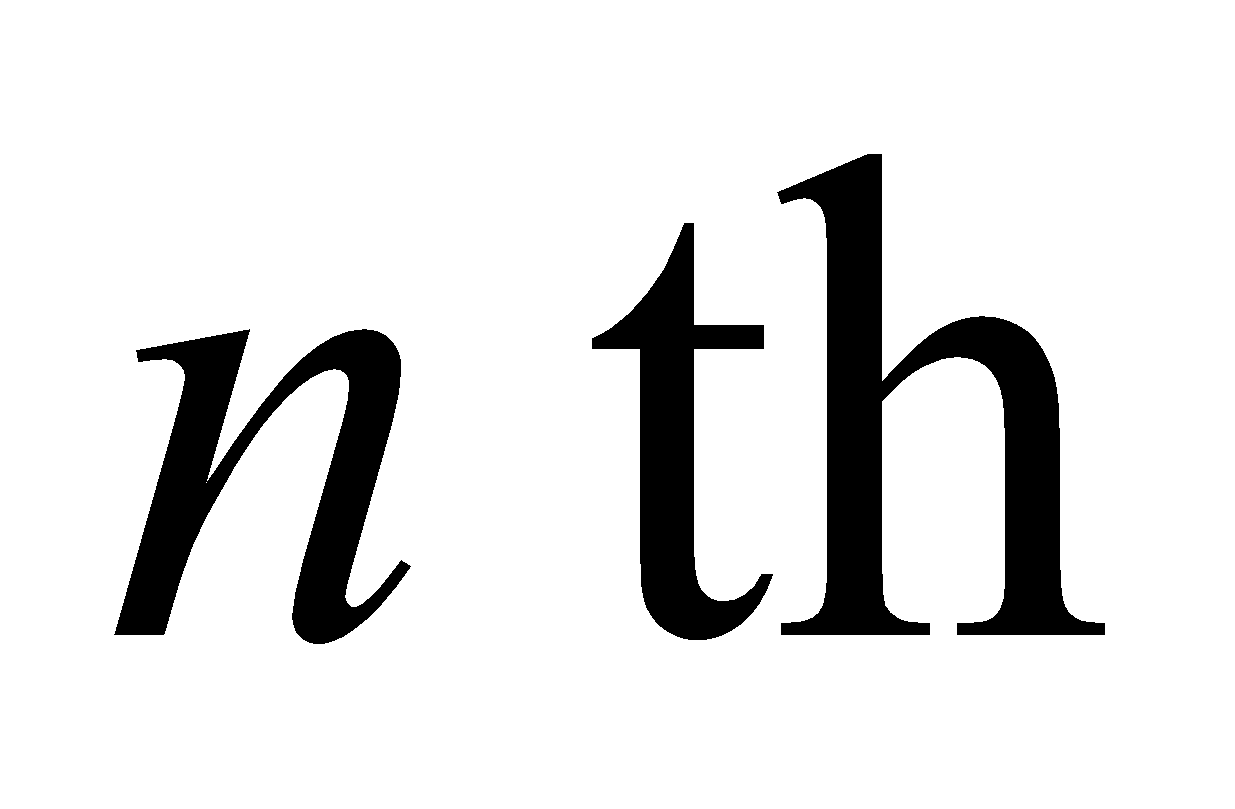Catalan number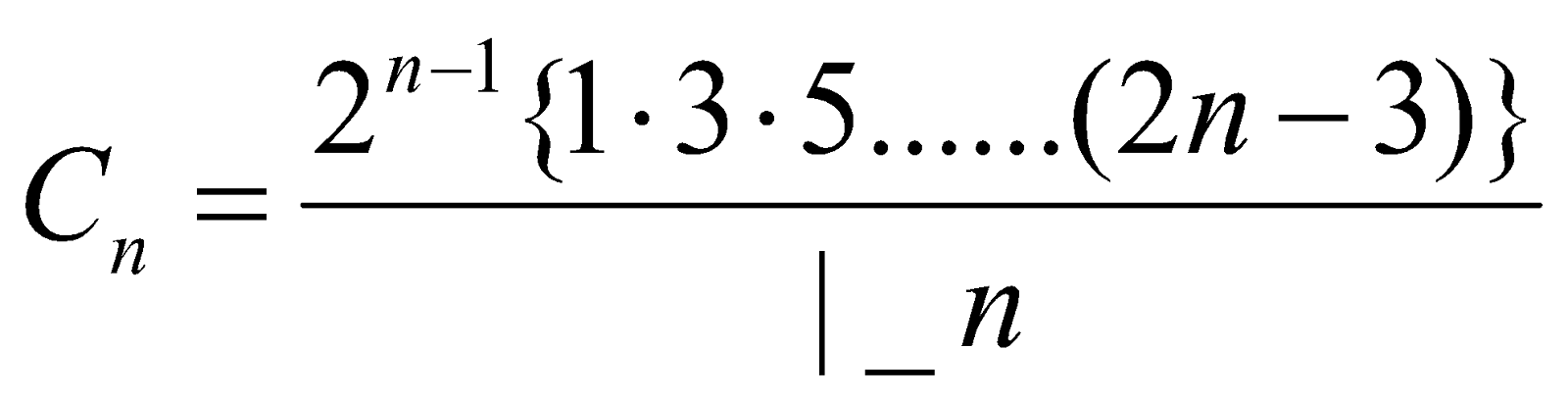4. (a) State the pigeonhole principle. 1
(b) How many integers between 100 and 700 are divisible by 3 or 5? 3
(c) Prove that given any 12 natural numbers we can choose 2 of them such that their difference is divisible by 11.        4
Or
Define binomial generating function. Find both binomial and exponential generating functions for the sequence 2, 2, 2, 2, ....

(B) Analysis – III (Complex Analysis)
(Marks: 45)
5. (a) State the condition under which a function is said to be analytic. 1
(b) Define harmonic function. Show that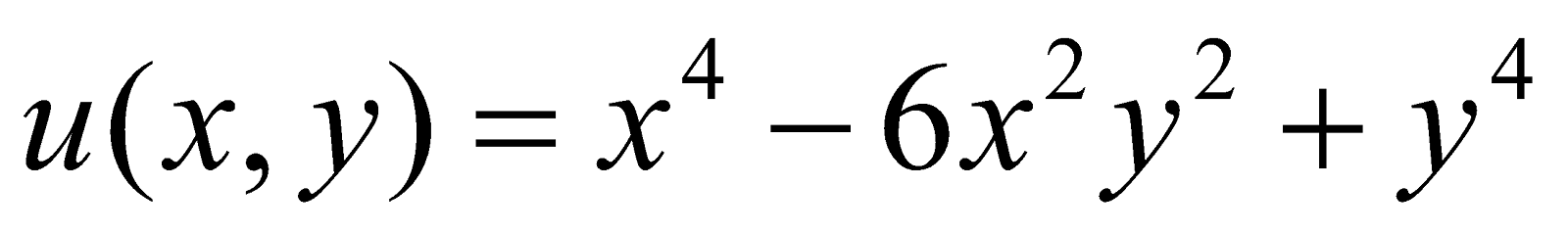is harmonic. 3
(c) State and prove the necessary conditions for a function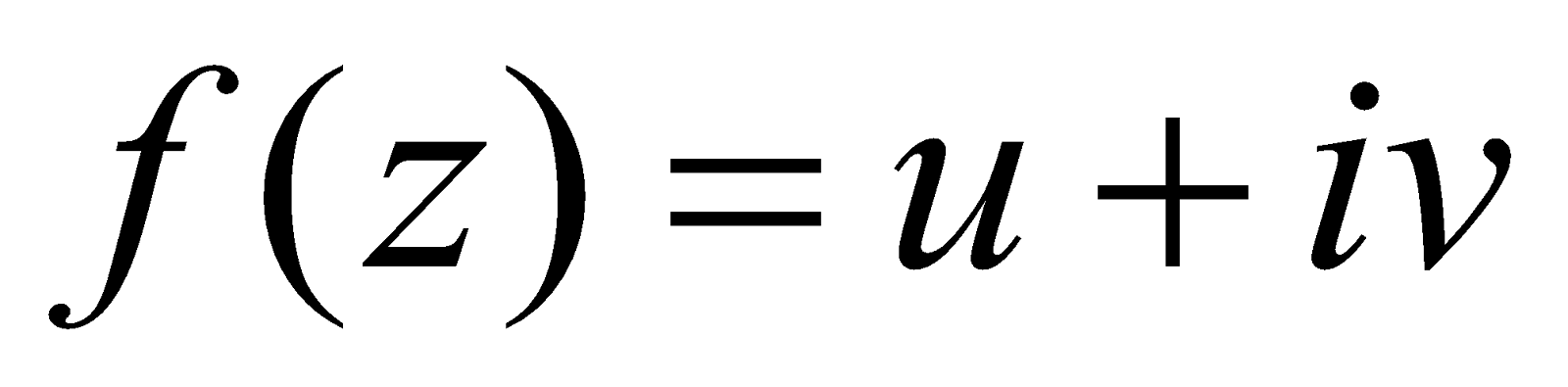to be analytic at all points in a region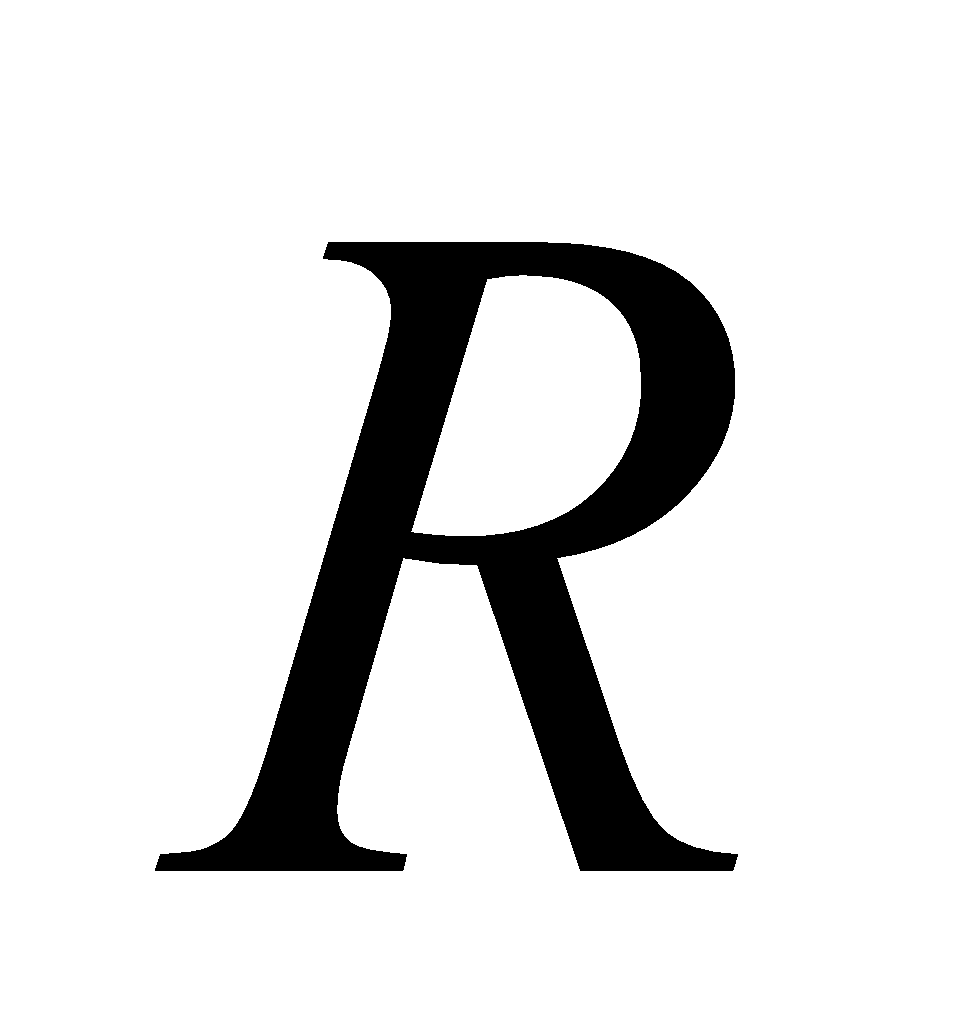.      6
Or
Show that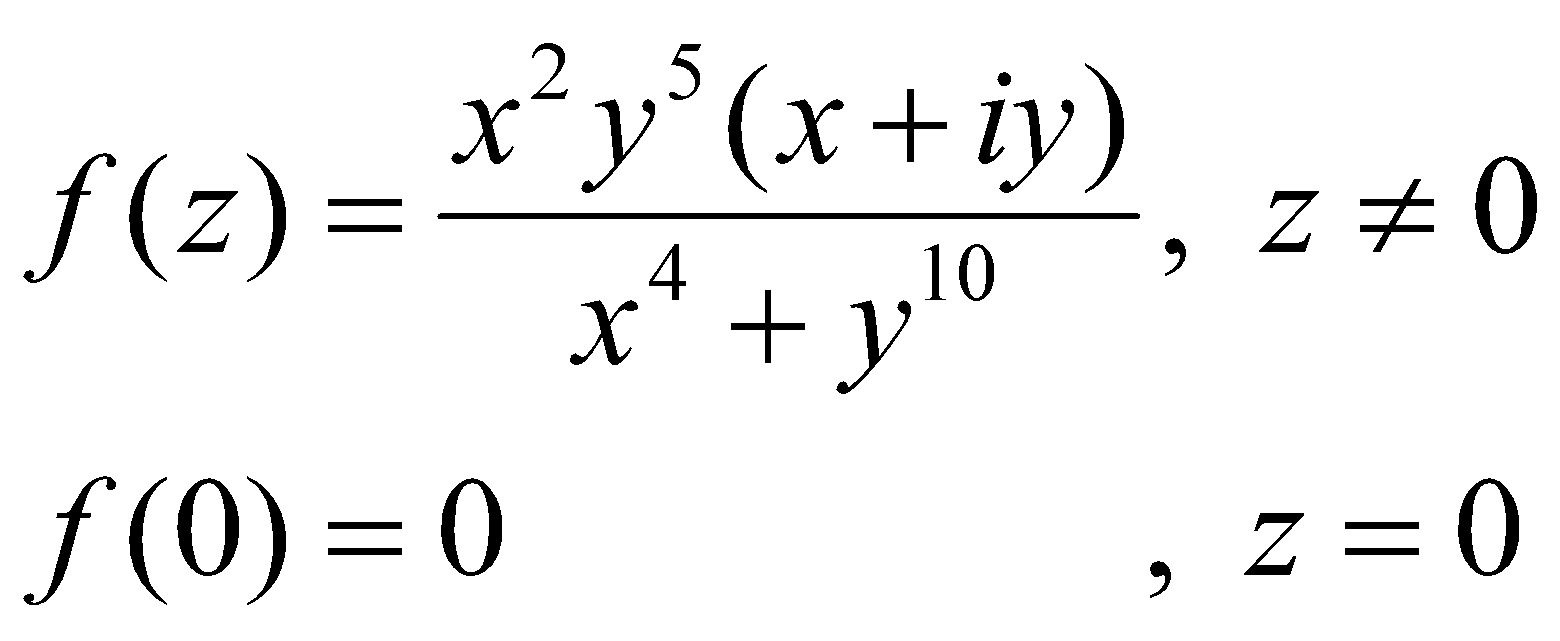is not analytic at the origin, although Cauchy-Riemann equations are satisfied. What is your opinion in this case?
6. (a) Define Jordan’s arc.
(b) Find the value of the integral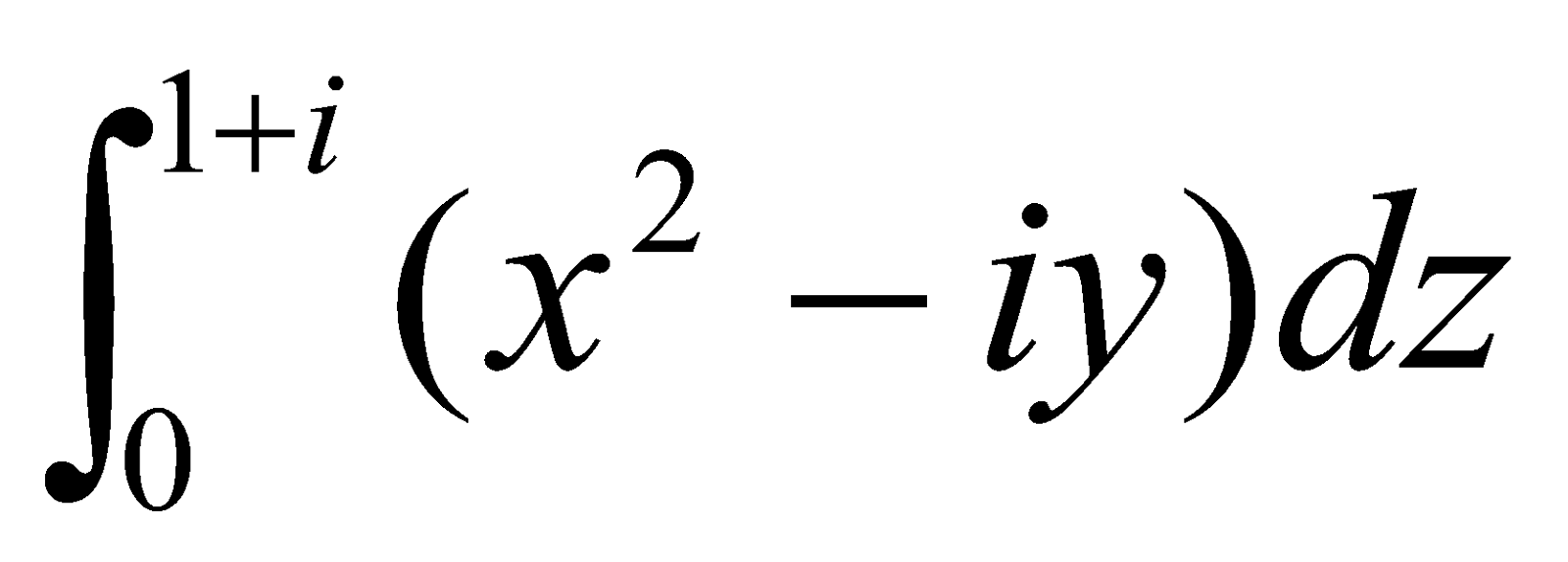where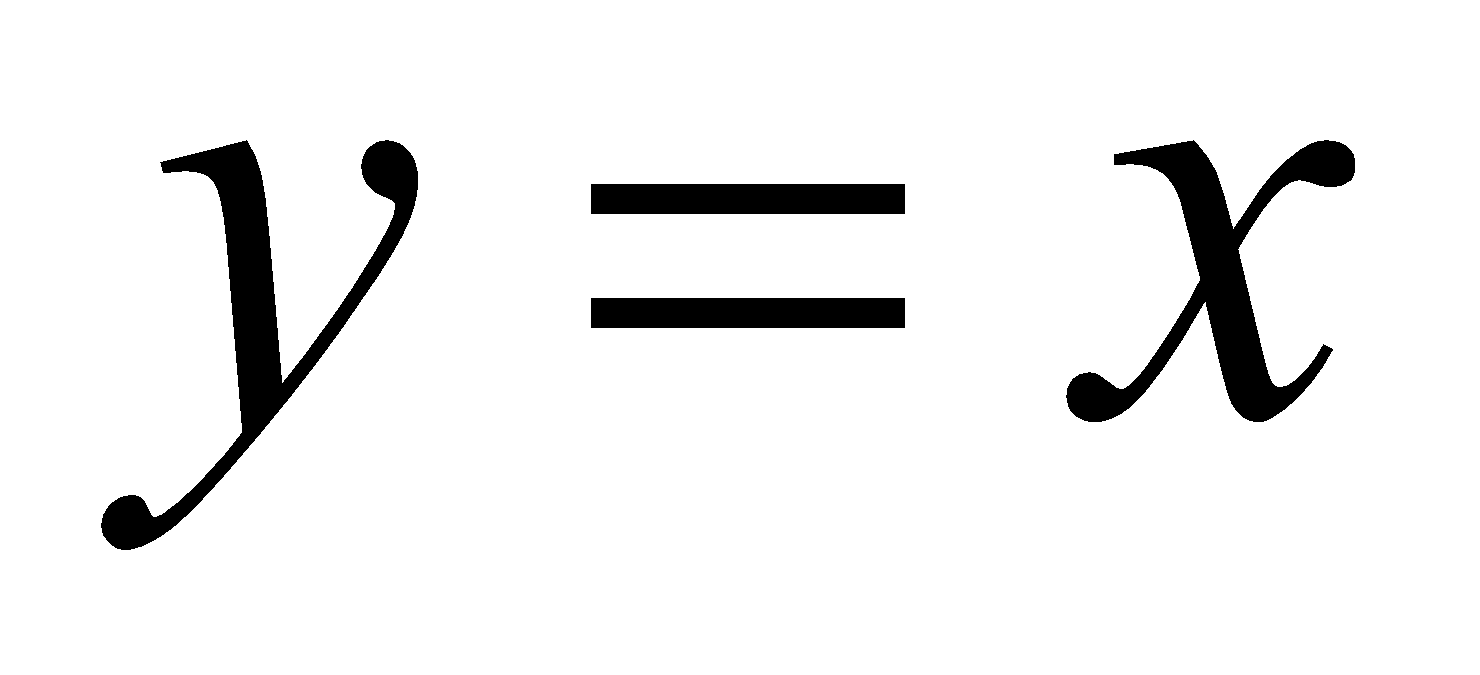.
(c) State and prove Cauchy’s integral theorem.
(d) If a function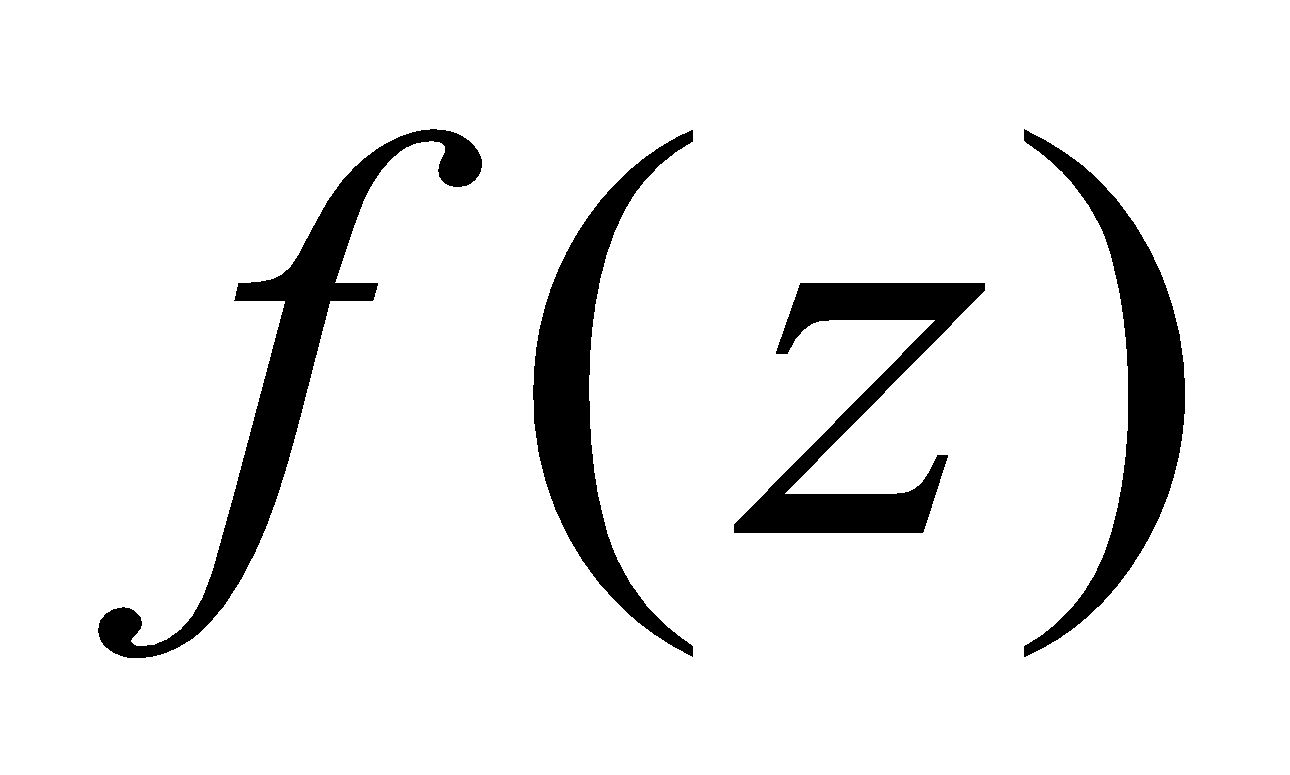is analytic for all finite values of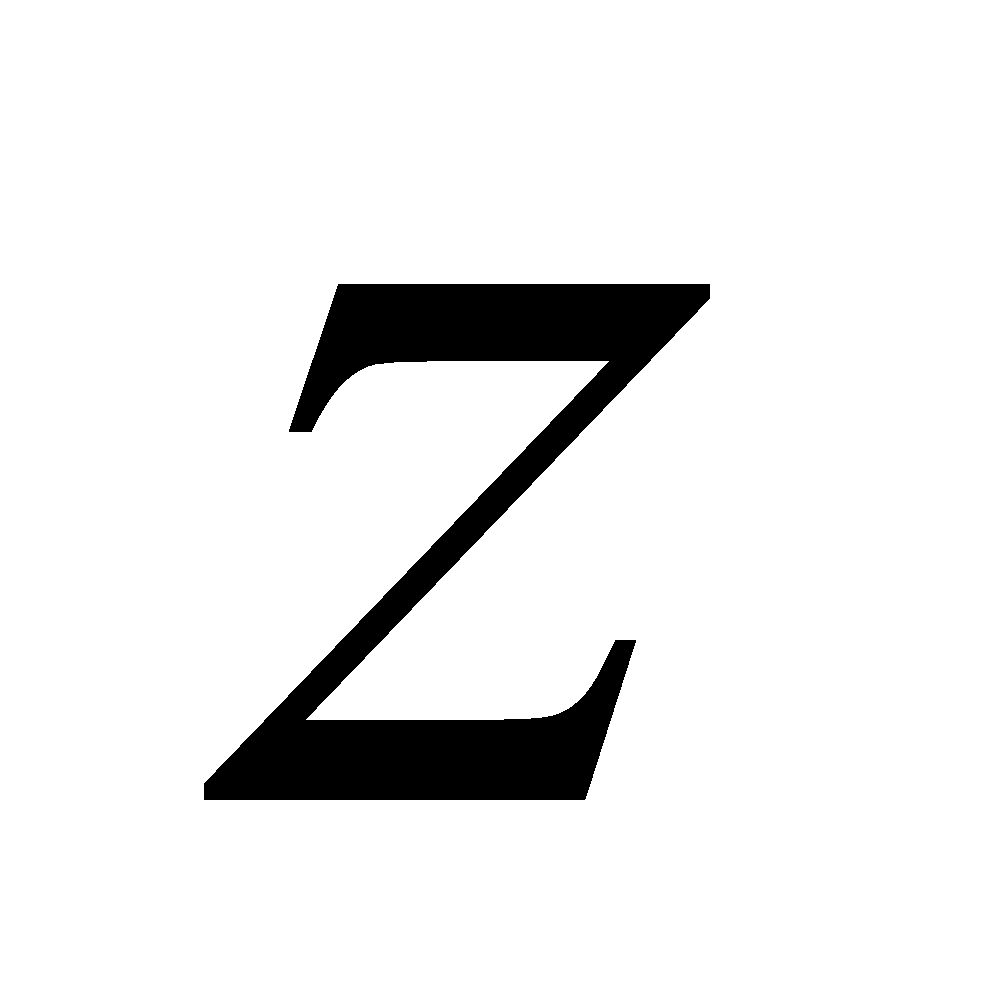and is bounded, then show that it is constant. 6
Or
Evaluate:
1.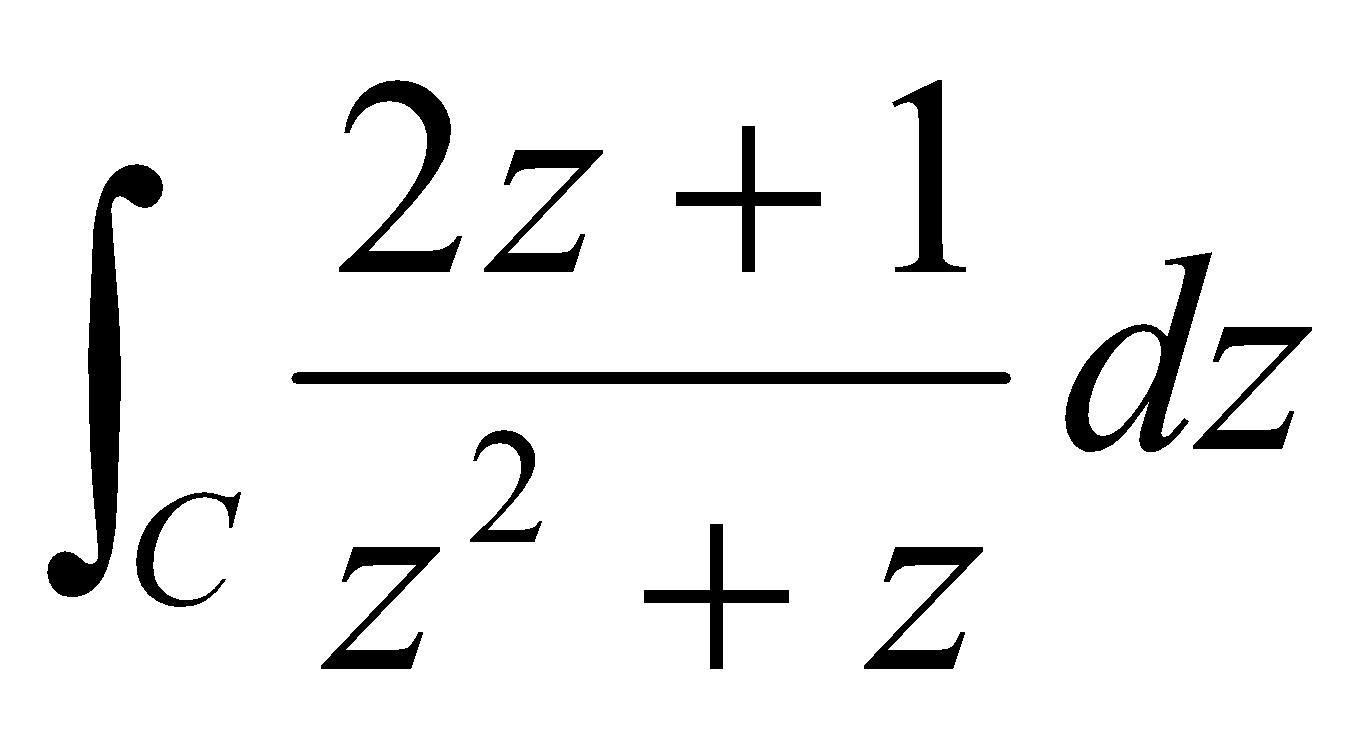, where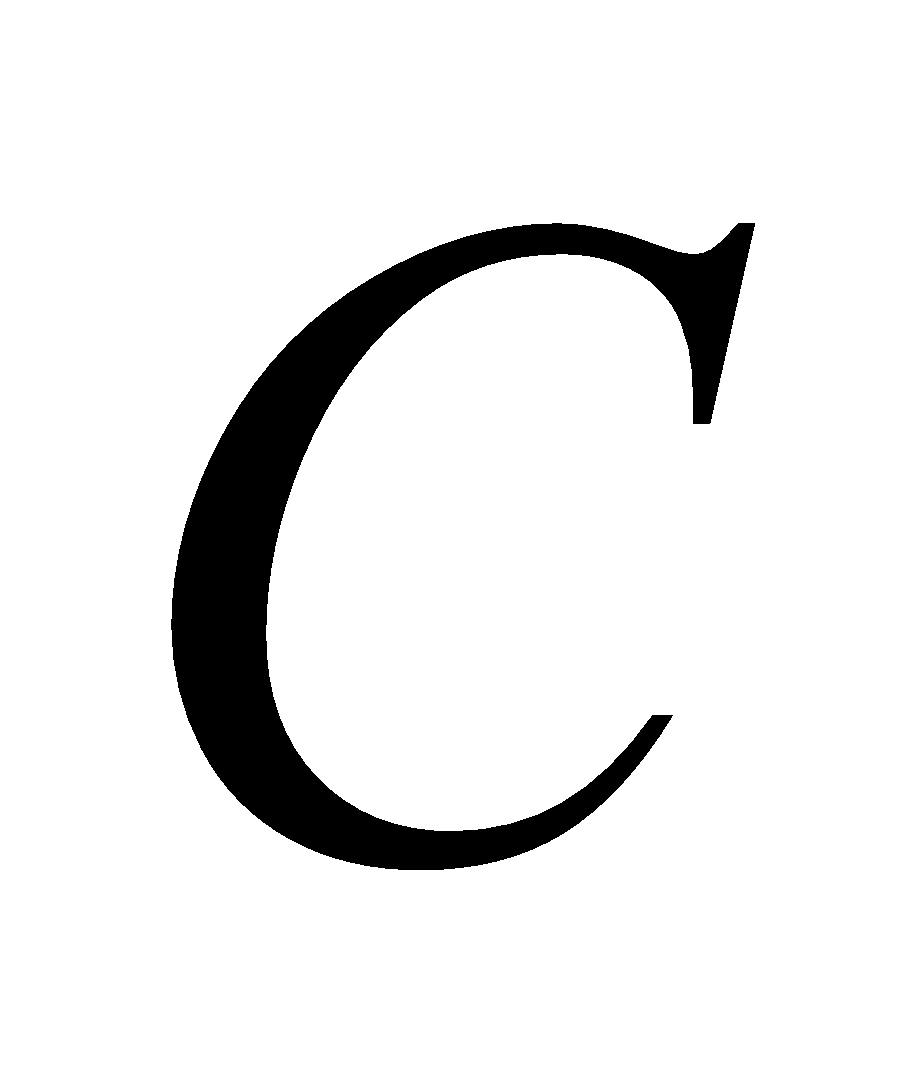is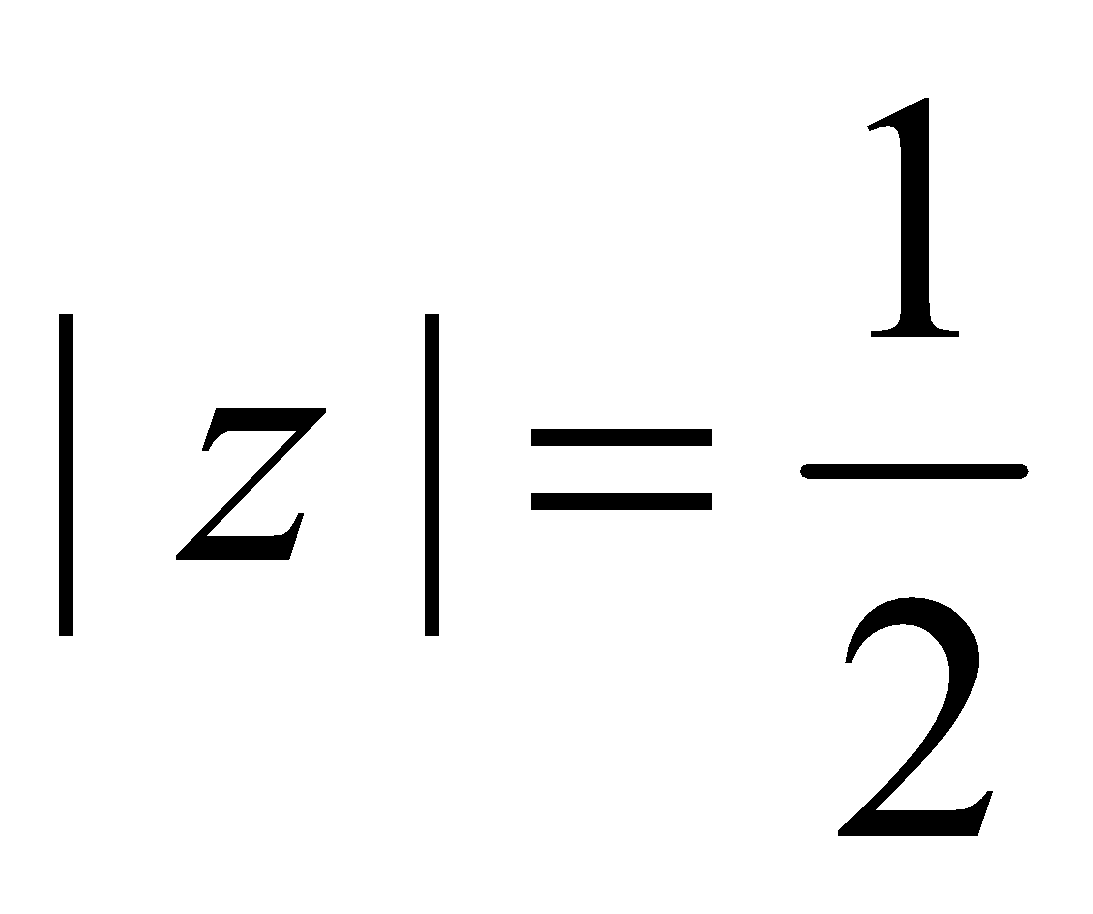2.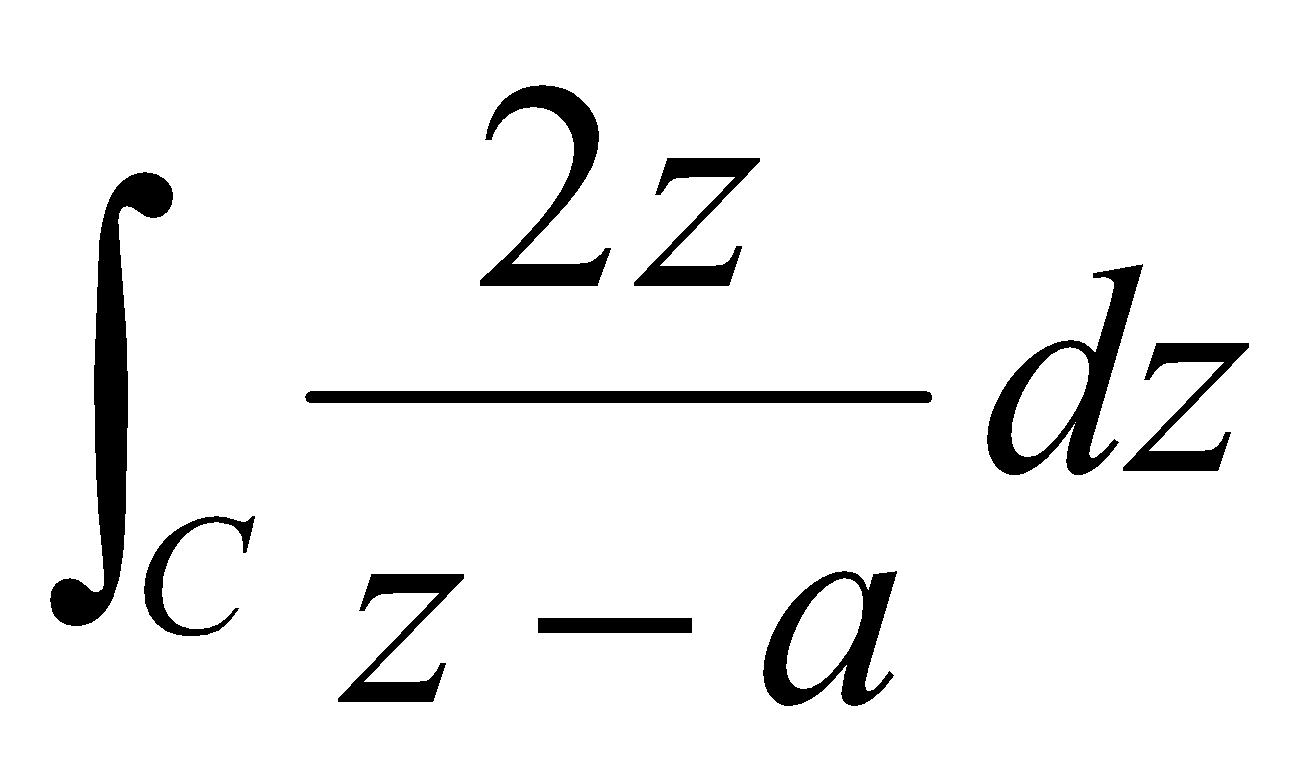, whereis7. (a) State and prove Taylor’s series. 1+5=6
(b) Expand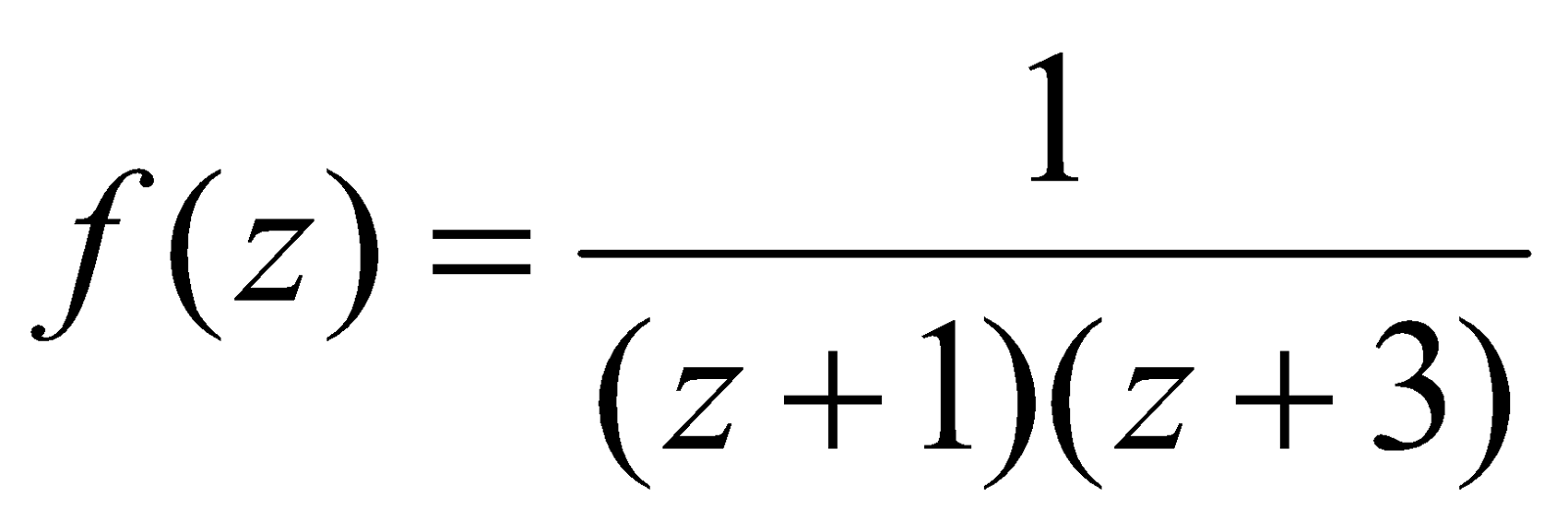is Laurent’s series, where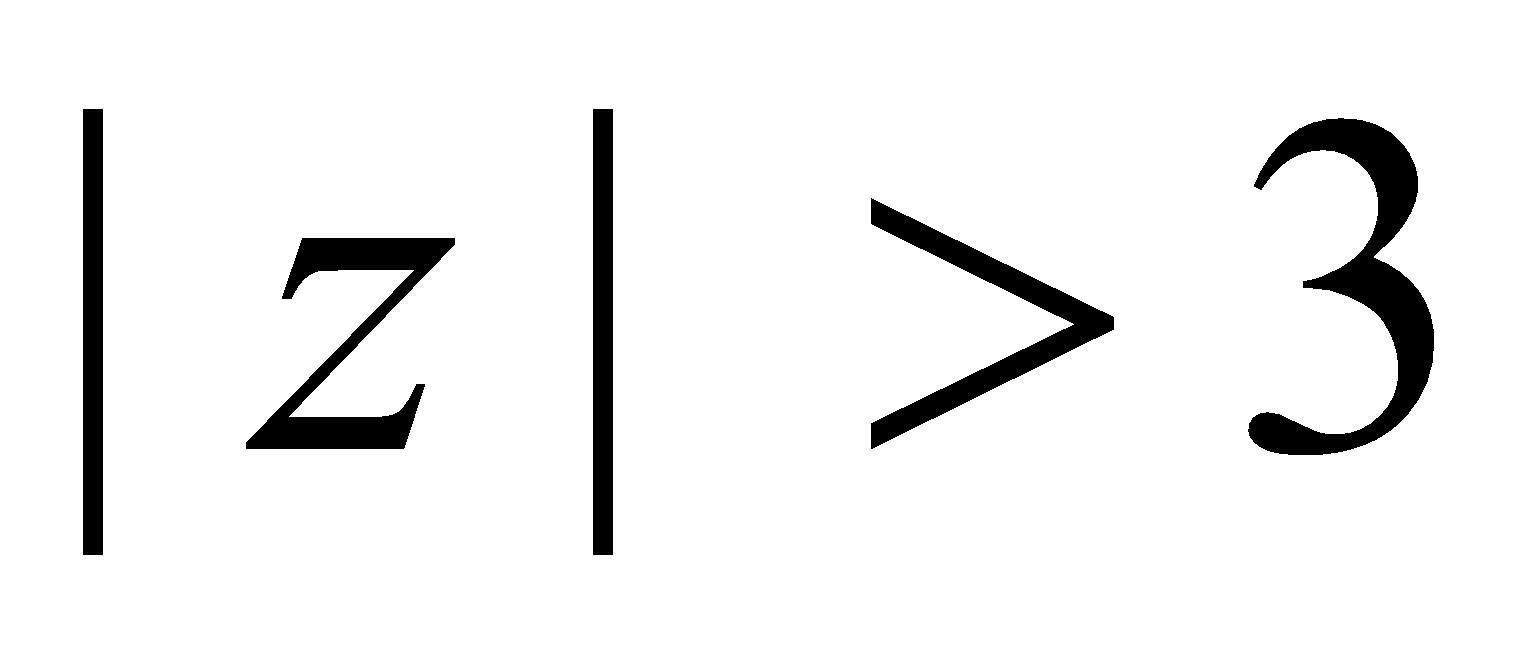. 2
8. (a) Define an isolated singular point of a function. 1
(b) Discuss the singularity of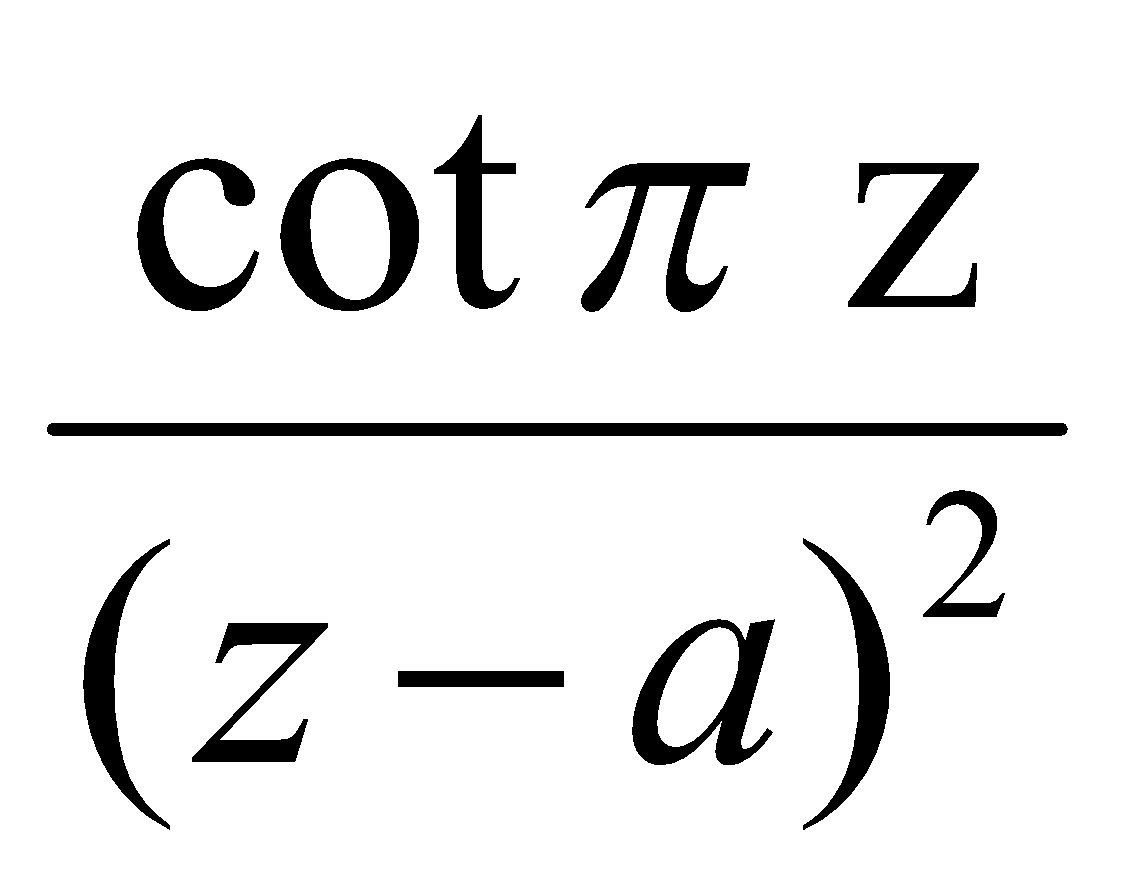at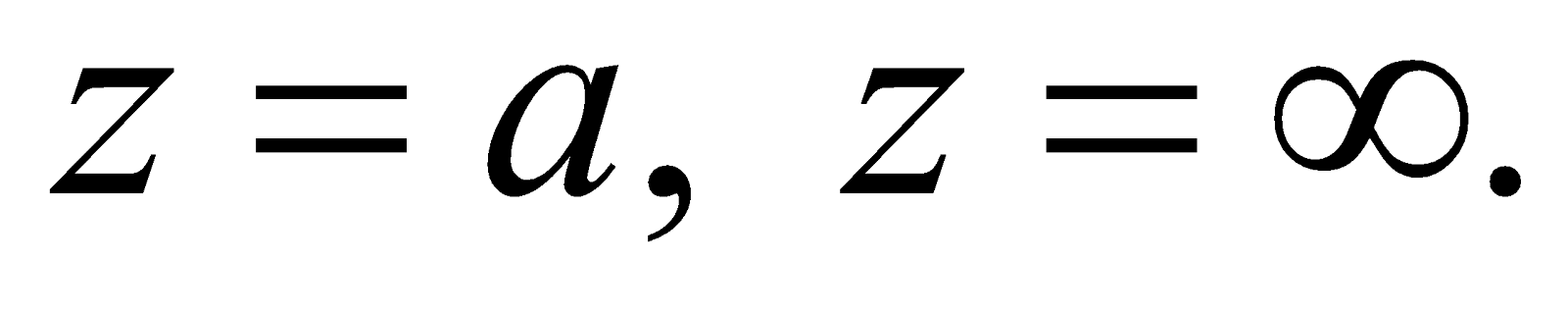2
(c) Evaluate (any two): 5x2=10
1.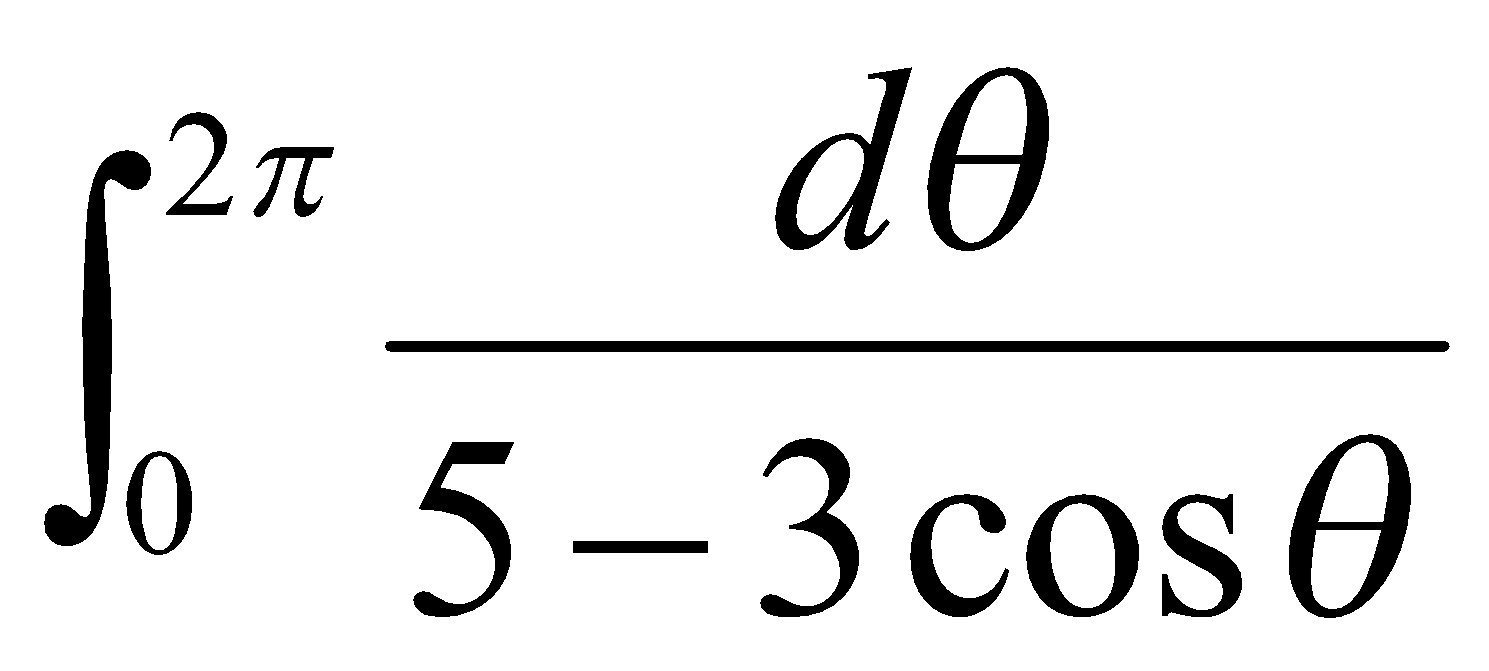2.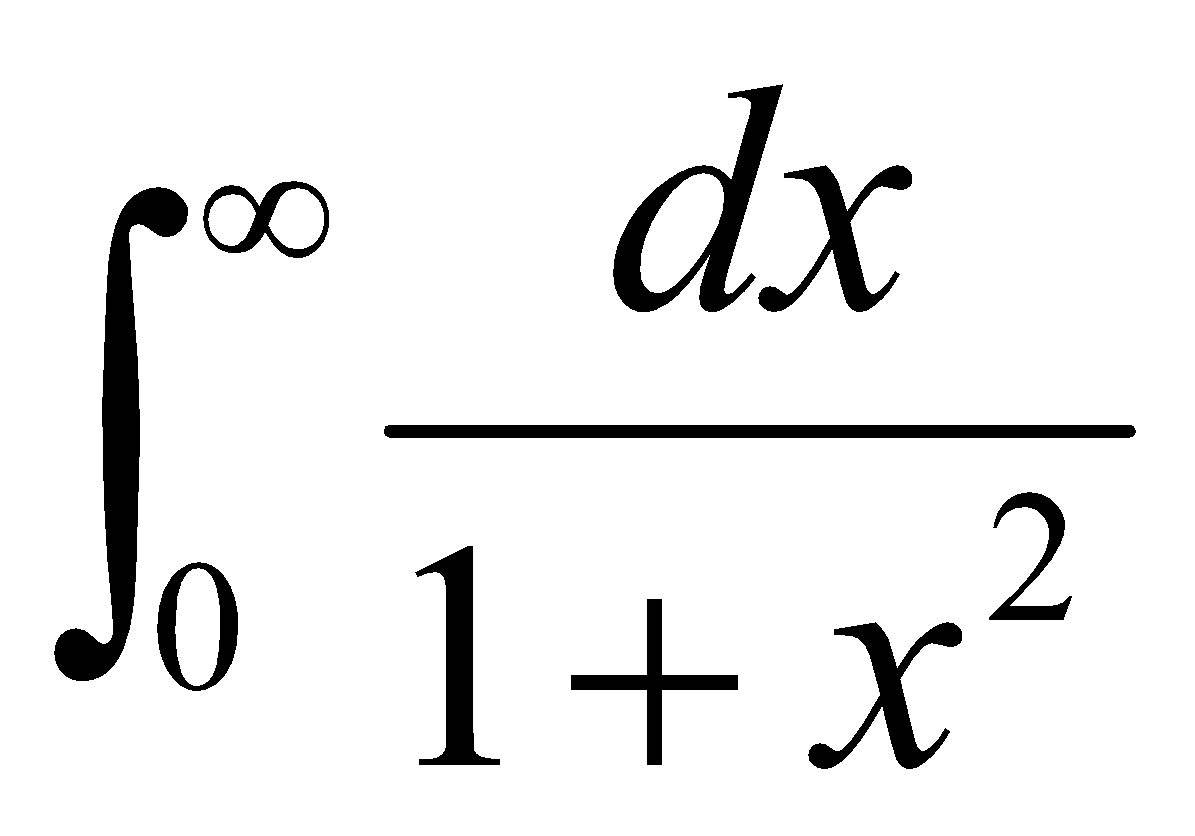3.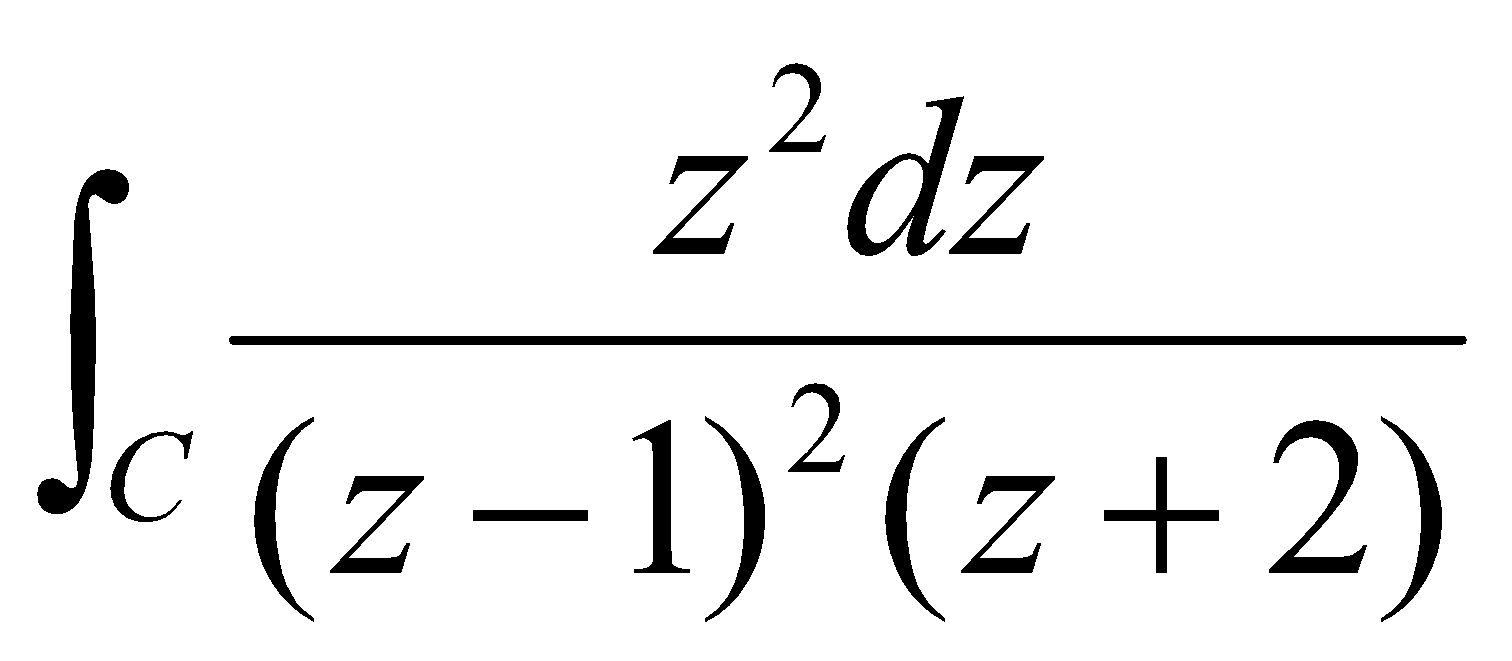, whereis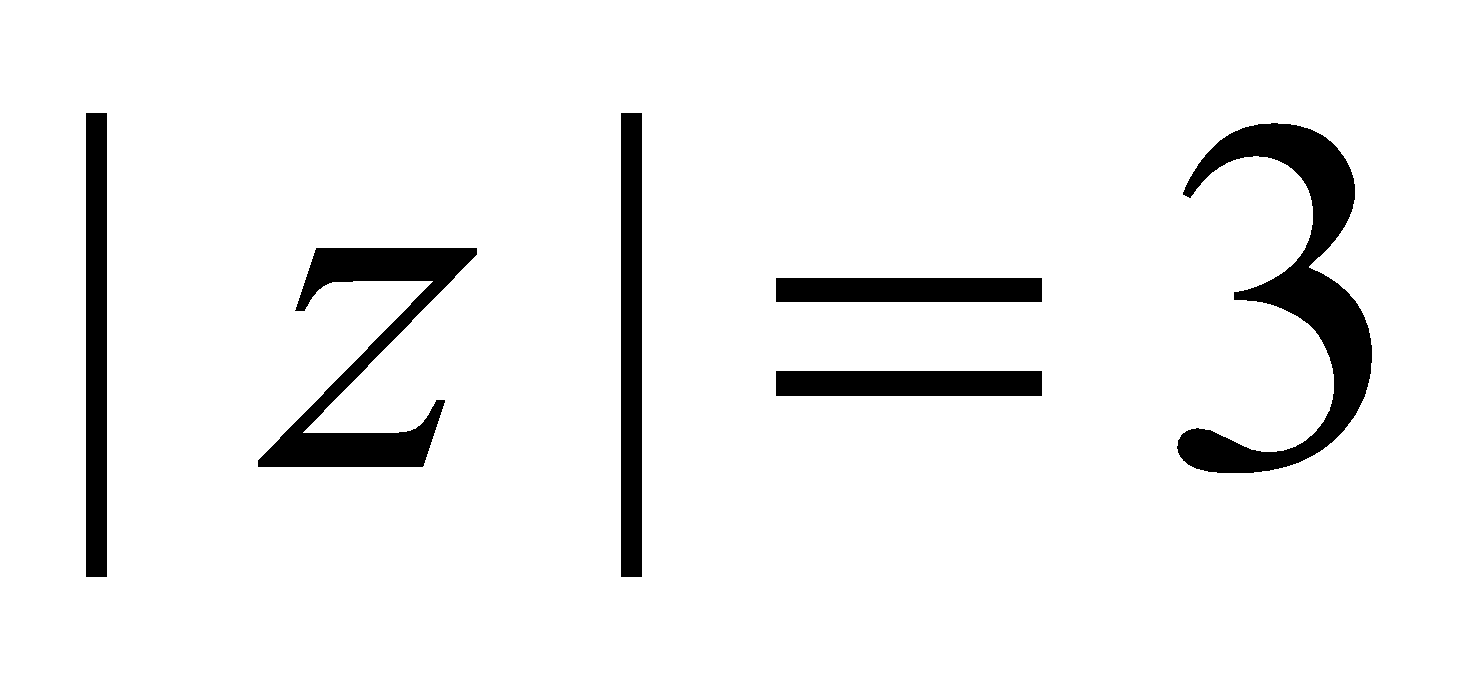4.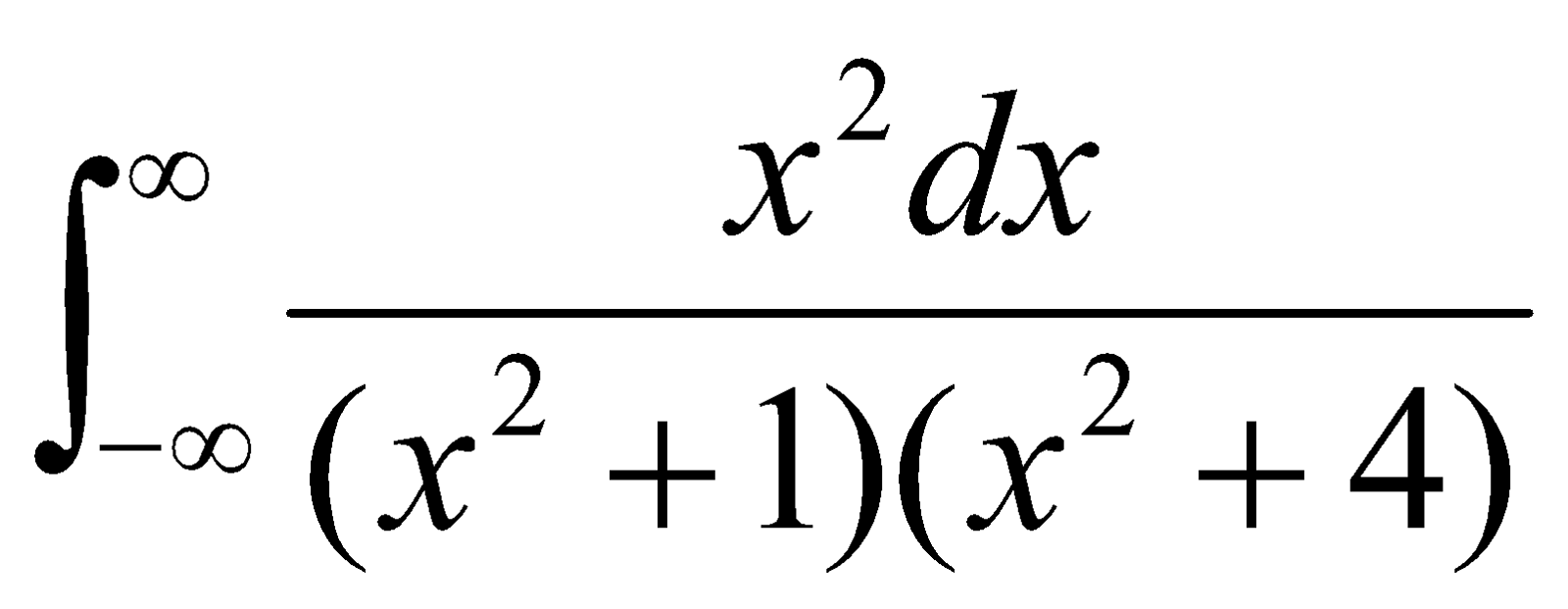***